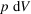International
Tables for
Crystallography
Volume D
Physical properties of crystals
Edited by A. Authier

International Tables for Crystallography (2006). Vol. D, ch. 1.1, p. 3

## Section 1.1.1.1. Notion of extensive and intensive quantities

A. Authiera*

aInstitut de Minéralogie et de la Physique des Milieux Condensés, Bâtiment 7, 140 rue de Lourmel, 75015 Paris, France
Correspondence e-mail: aauthier@wanadoo.fr

#### 1.1.1.1. Notion of extensive and intensive quantities

| top | pdf |

Physical laws express in general the response of a medium to a certain influence. Most physical properties may therefore be defined by a relation coupling two or more measurable quantities. For instance, the specific heat characterizes the relation between a variation of temperature and a variation of entropy at a given temperature in a given medium, the dielectric susceptibility the relation between electric field and electric polarization, the elastic constants the relation between an applied stress and the resulting strain etc. These relations are between quantities of the same nature: thermal, electrical and mechanical, respectively. But there are also cross effects, for instance:

 (a) thermal expansion and piezocalorific effect: mechanical reaction to a thermal impetus or the reverse; (b) pyroelectricity and electrocalorific effect: electrical response to a thermal impetus or the reverse; (c) piezoelectricity and electrostriction: electric response to a mechanical impetus; (d) piezomagnetism and magnetostriction: magnetic response to a mechanical impetus; (e) photoelasticity: birefringence produced by stress; (f) acousto-optic effect: birefringence produced by an acoustic wave; (g) electro-optic effect: birefringence produced by an electric field; (h) magneto-optic effect: appearance of a rotatory polarization under the influence of a magnetic field.

The physical quantities that are involved in these relations can be divided into two categories:

• (i) extensive quantities, which are proportional to the volume of matter or to the mass, that is to the number of molecules in the medium, for instance entropy, energy, quantity of electricity etc. One uses frequently specific extensive parameters, which are given per unit mass or per unit volume, such as the specific mass, the electric polarization (dipole moment per unit volume) etc.

• (ii) intensive parameters, quantities whose product with an extensive quantity is homogeneous to an energy. For instance, volume is an extensive quantity; the energy stored by a gas undergoing a change of volume dV under pressure p is. Pressure is therefore the intensive parameter associated with volume. Table 1.1.1.1gives examples of extensive quantities and of the related intensive parameters.

 Table 1.1.1.1| top | pdf | Extensive quantities and associated intensive parameters
 The last four lines of the table refer to properties that are time dependent.
Extensive quantitiesIntensive parameters
Volume Pressure
Strain Stress
Displacement Force
Entropy Temperature
Quantity of electricity Electric potential
Electric polarization Electric field
Electric displacement Electric field
Magnetization Magnetic field
Magnetic induction Magnetic field
Reaction rate Chemical potential
Heat flow Temperature gradient
Diffusion of matter Concentration gradient
Electric current Potential gradient